# NCERT Solutions class 12 Chemistry Solutions Part 1## myCBSEguide App

CBSE, NCERT, JEE Main, NEET-UG, NDA, Exam Papers, Question Bank, NCERT Solutions, Exemplars, Revision Notes, Free Videos, MCQ Tests & more.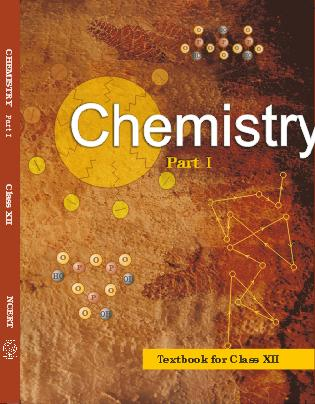## NCERT Class 12 Chemistry Chapter-wise Solutions

•  1 – The Solid State
• 2 – Solutions
• 3 – Electrochemistry
• 4 – Chemical Kinetics
• 5 – Surface Chemistry
• 6 – General Principles and Processes of Isolation of Elements
• 7 – The p-Block Elements
• 8 – The d-and f-Blocks Elements
• 9 – Coordination Compounds
• 10 – Haloalkanes and Haloarenes
• 11 – Alcohols, Phenols and Ethers
• 12 – Aldehydes, Ketones and Carboxylic Acids
• 13 – Amines
• 14 – Biomolecules
• 15 – Polymers
• 16 – Chemistry in Everyday Life

### CHAPTER TWO SOLUTIONS

• 2.1 Types of Solutions
• 2.2 Expressing Concentration of Solutions
• 2.3 Solubility
• 2.4 Vapour Pressure of Liquid Solutions
• 2.5 Ideal and Non-Ideal Solutions
• 2.6 Colligative Properties and Determination of Molar Mass
• 2.7 Abnormal Molar Masses

## NCERT Solutions class 12 Chemistry Solutions Part 1

1. Define the term solution. How many types of solutions are formed? Write briefly about each type with an example.

2. Give an example of solid solution in which the solute is a gas.

3. Define the following terms:

(i) Mole fraction

(ii) Molality

(iii) Molarity

(iv) Mass percentage.

4. Concentrated nitric acid used in laboratory work is 68% nitric acid by mass in aqueous solution. What should be the molarity of such a sample of the acid if the density of the solution is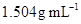?

Molarity of solution =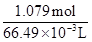= 16.23 M

5. A solution of glucose in water is labelled as 10% w/w, what would be the molality and mole fraction of each component in the solution? If the density of solution is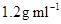, then what shall be the molarity of the solution?

6. How many mL of 0.1 M HCl are required to react completely with 1 g mixture of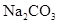and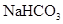containing equimolar amounts of both?

7. A solution is obtained by mixing 300g of 25% solution and 400 g of 40% solution by mass. Calculate the mass percentage of the resulting solution.

8. An antifreeze solution is prepared from 222.6 g of ethylene glycol (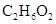) and 200 g of water. Calculate the molality of the solution. If the density of the solution is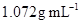, then what shall be the molarity of the solution?

9. A sample of drinking water was found to be severely contaminated with chloroform (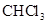) supposed to be a carcinogen. The level of contamination was 15 ppm (by mass):

(i) express this in percent by mass

(ii) determine the molality of chloroform in the water sample.

10. What role does the molecular interaction play in a solution of alcohol and water?

11. Why do gases always tend to be less soluble in liquids as the temperature is raised?

12. State Henry’s law and mention some important applications?

13. The partial pressure of ethane over a solution containing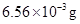of ethane is 1 bar. If the solution contains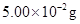of ethane, then what shall be the partial pressure of the gas?

4. What is meant by positive and negative deviations from Raoult’s law and how is the sign of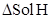related to positive and negative deviations from Raoult’s law?

15. An aqueous solution of 2% non-volatile solute exerts a pressure of 1.004 bar at the normal boiling point of the solvent. What is the molar mass of the solute?

16. Heptane and octane form an ideal solution. At 373 K, the vapour pressures of the two liquid components are 105.2 kPa and 46.8 kPa respectively. What will be the vapour pressure of a mixture of 26.0 g of heptane and 35 g of octane?

17. The vapour pressure of water is 12.3 kPa at 300 K. Calculate vapour pressure of 1 molal solution of a non-volatile solute in it.

18. Calculate the mass of a non-volatile solute (molar mass 40 g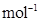) which should be dissolved in 114 g octane to reduce its vapour pressure to 80%.

19. A solution containing 30 g of non-volatile solute exactly in 90 g of water has a vapour pressure of 2.8 kPa at 298 K. Further, 18 g of water is then added to the solution and the new vapour pressure becomes 2.9 kPa at 298 K. Calculate:

i. molar mass of the solute

ii. vapour pressure of water at 298 K.

20. A 5% solution (by mass) of cane sugar in water has freezing point of 271 K. Calculate the freezing point of 5% glucose in water if freezing point of pure water is 273.15 K.

## NCERT Solutions class 12 Chemistry

NCERT Solutions Class 12 Chemistry PDF (Download) Free from myCBSEguide app and myCBSEguide website. Ncert solution class 12 Chemistry includes text book solutions from both part 1 and part 2. NCERT Solutions for CBSE Class 12 Chemistry have total 16 chapters. 12 Chemistry NCERT Solutions in PDF for free Download on our website. Ncert Chemistry class 12 solutions PDF and Chemistry ncert class 12 PDF solutions with latest modifications and as per the latest CBSE syllabus are only available in myCBSEguide

To download NCERT Solutions for class 12 Chemistry, Physics, Biology, History, Political Science, Economics, Geography, Computer Science, Home Science, Accountancy, Business Studies and Home Science; do check myCBSEguide app or website. myCBSEguide provides sample papers with solution, test papers for chapter-wise practice, NCERT solutions, NCERT Exemplar solutions, quick revision notes for ready reference, CBSE guess papers and CBSE important question papers. Sample Paper all are made available through the best app for CBSE students and myCBSEguide website